Disclosure: This post may contain affiliate links, meaning when you click the links and make a purchase, we receive a commission.

# Find difference between two numbers (positive or negative) in Excel

Want to know how to get the difference between two numbers (positive or negative) in Excel? Or how to calculate the percentage difference for negative numbers in Excel?

You’re in the right place.

Let’s continue…

## Subtraction definition and nomenclature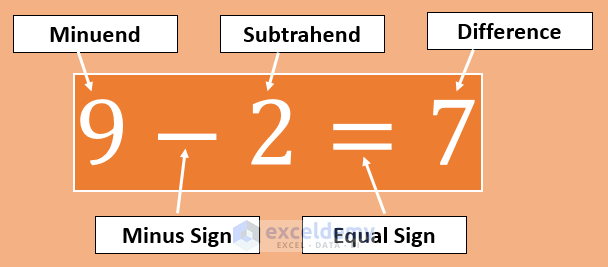The subtraction operation has the following participants:

• Minuend: A quantity or number from which another is to be subtracted. In the above example, 9 is the minuend.
• Minus Sign (-): Then we use a minus symbol (-) to find the difference between two numbers.
• Subtrahend: Subtrahend is the quantity or number to be subtracted from minuend.
• Equal Sign (=): Then we place an equal sign (=).
• Difference: Difference is the result of the subtract operation.

## How to find the difference between two numbers in Excel

In MS Excel, you will not find any SUBTRACT function to perform the subtraction operation. Instead, we use the regular minus (-) symbol to perform subtractions.

### 1) Using numbers directly in the formula

In this way, we input the numbers directly in the formula. You know, every formula starts with an equal sign in Excel

So, input an equal sign (=) to start an Excel formula > Input the minuend value > Input the minus sign (-) > Place the subtrahend value > Press Enter

Example: =50-5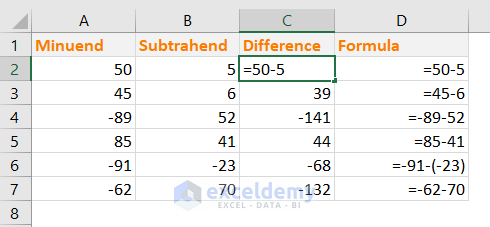Note: If the subtrahend value is negative, use the parentheses to place the number in the subtraction formula like this: =-91-(-23)

Why is this method not suggested?

• If you have more than one subtraction, you have to write a formula for every subtraction individually
• You cannot copy the same formula for another set of numbers
• Time-consuming as you have to write a formula for every set of numbers individually

### 2) Using cell references instead of numbers in the formula

This is more effective and time-saving. We can make a formula for a single set of numbers and then use the formula for other cells.

So, we shall use this method and this is why Excel is so useful.

• In the cell C2, input this formula: =A2-B2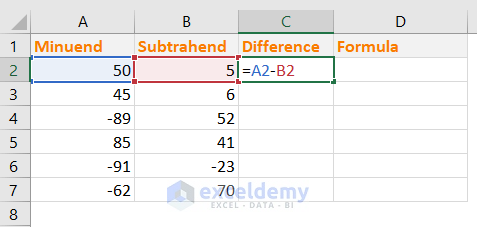• Press Enter and this is the result we get.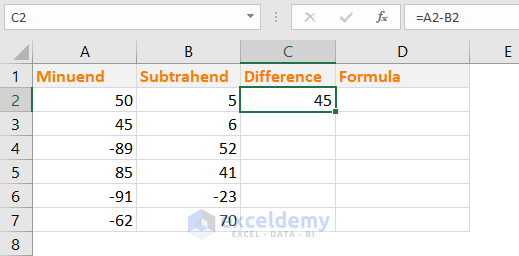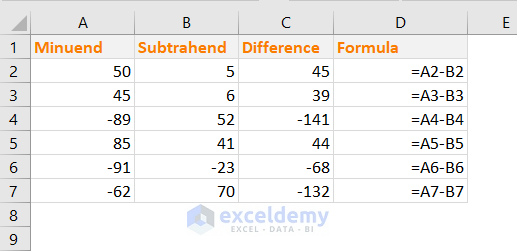### 3) Calculate the absolute difference between two numbers in Excel (Using ABS() function)

To get the absolute difference between two numbers in Excel, we shall use the ABS() Excel function.

ABS() function returns the absolute value of a number, a number without its sign.

Syntax of ABS Function: ABS(number)

We have used the ABS() Function in the same data. You see that the previous negative differences are now showing as positive numbers (without any sign).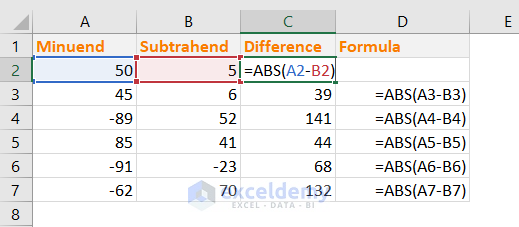## The percentage difference between the two numbers in Excel

Percent Difference and Percent Error are actually the same things. In Percent Difference, you will compare two experimental values.

This is the equation to calculate the Percent Difference.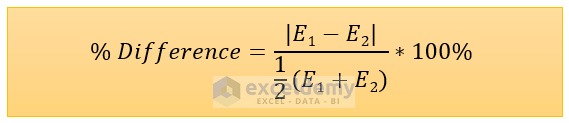Here, E1 = First experimental value and E2 = Second experimental value

Note: Percentage Change ((New value/Old value) -1) and Percent Difference are two different phenomena in Physics.

In Excel, we can easily calculate the Percent Difference: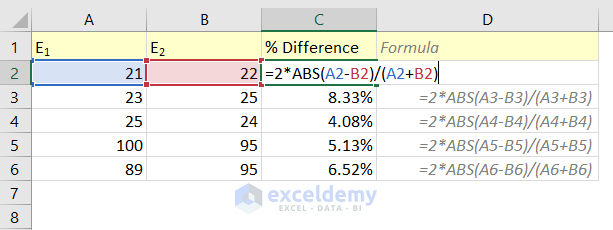We have used this formula in the cell C2: =2*ABS(A2-B2)/(A2+B2)

How does this formula work?

• The difference between number A2 and B2 (A2-B2) can be negative. So, we have used the ABS() function (ABS(A2-B2)) to make the number absolute.
• Then we have multiplied the absolute value by 2 and then divided the value by (A2+B2)

## Calculate Percentage Change for negative numbers in Excel

Theoretically and even practically, you cannot find the percentage change for negative numbers. When it is not possible theoretically, how can we calculate them in Excel?

Not possible. Whatever methods you use, you will find misleading results.

Here I will show 3 methods to calculate the percentage change of negative numbers in Excel but all of them will mislead you. So, be aware of it before using them in your work.

### 1) Calculate the % change of negative numbers by making the denominator absolute

Say, you want to calculate the percentage of two values:

Old value: -400

New value: 200

We know, % change = ((New value – Old value )/ Old value) x 100%

If we apply this formula to calculate the % change of the two above values (-400 & 200), this will be the result:

= ((200 – (-400))/-400)*100%

= 600/(-400)*100%

= -150%

This is the completely wrong answer.

As in this way, you don’t get the correct answer, so some companies use the ABS method. In this method, the denominator is made absolute.

In the following image, I have shown the ABS denominator method.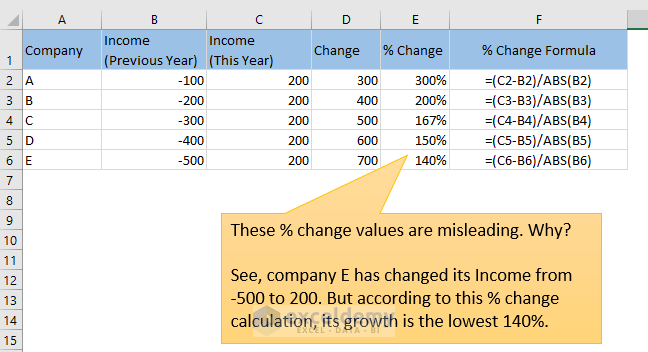These results are also misleading because you see -100 to 200 shows the biggest % change when -500 to 200 shows the smallest % change.

Take a look at the following image: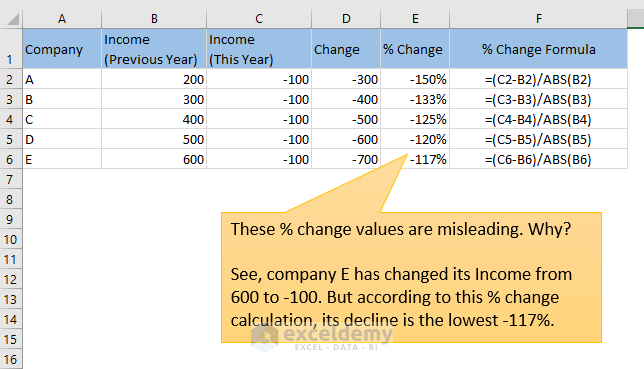You see, though company E has seen the sharpest decline in income (from 600 to -100), the % change is showing that it has declined the lowest (-117%).

### 2) Shift the numbers to make them positive

This is a tricky method but the result is not acceptable.

Say, we have two values:

Old value: -50

New value: 20

We will shift these two numbers using their absolute plus and then multiplying it by 2: (|-50|+ |20|)*2 = 140

shifted_old value = -50 + 140 = 90

shifted_new value = 20 + 140 = 160

Now, we shall calculate their % change: ((160-90)/90)*100% = 77.78%

Let’s see whether this method gives us satisfactory results: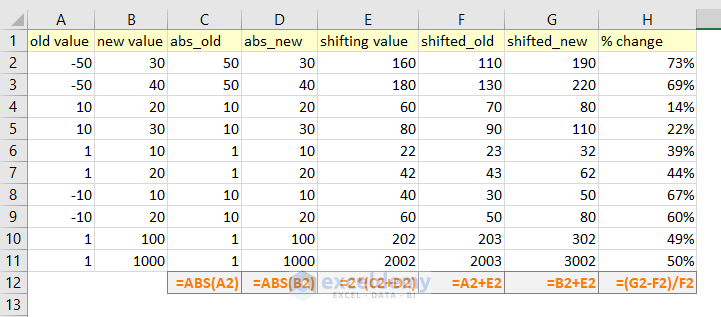Just check out the last two rows: % change from 1 to 100 and 1 to 1000 are almost same.

But they should be: ((100-1)/1)*100% = 99% and ((1000-1)/1)*100% = 999%

Whatever methods you use, you will get no correct results.

So, what will be the solution?

Solution: Show N/A (or anything) when the % change of negative numbers appears

We shall use this formula:

`IF(MIN(old_value, new_value)<=0, "N/A", (new_value-old_value)/old_value)`

Check out the image below. When any of the two values is negative, the formula returns “N/A” value.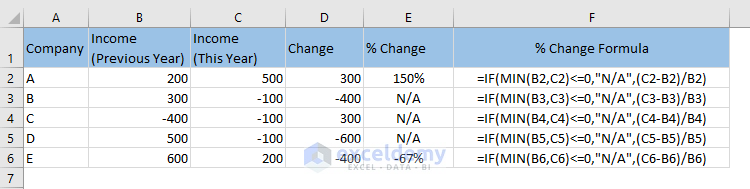1. Reply